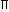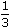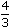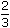# Aptitude :: Volume and Surface Area

1. CUBOID

Let length = l, breadth = b and height = h units. Then

1. Volume = (l x b x h) cubic units.

2. Surface area = 2(lb + bh + lh) sq. units.

3. Diagonal = l2 + b2 + h2 units.

2. CUBE

Let each edge of a cube be of length a. Then,

1. Volume = a3 cubic units.

2. Surface area = 6a2 sq. units.

3. Diagonal = 3a units.

3. CYLINDER

Let radius of base = r and Height (or length) = h. Then,

1. Volume = (r2h) cubic units.

2. Curved surface area = (2rh) sq. units.

3. Total surface area = 2r(h + r) sq. units.

4. CONE

Let radius of base = r and Height = h. Then,

1. Slant height, l = h2 + r2 units.

2. Volume =r2hcubic units.

3. Curved surface area = (rl) sq. units.

4. Total surface area = (rl +r2) sq. units.

5. SPHERE

Let the radius of the sphere be r. Then,

1. Volume =r3cubic units.

2. Surface area = (4r2) sq. units.

6. HEMISPHERE

Let the radius of a hemisphere be r. Then,

1. Volume =r3cubic units.

2. Curved surface area = (2r2) sq. units.

3. Total surface area = (3r2) sq. units.

Note: 1 litre = 1000 cm3.